# The ordered partial partition polytope

In the tensor rank paper we introduced a new family of axis-aligned n-dimensional polytopes, one for each positive integer n. The vertices are naturally identified with ordered partial partitions (OPPs) of {1, …, n}, and the edges correspond to converting one OPP into an ‘adjacent’ OPP by moving an element in a specific way.

Here are the polytopes for n = 2 and n = 3: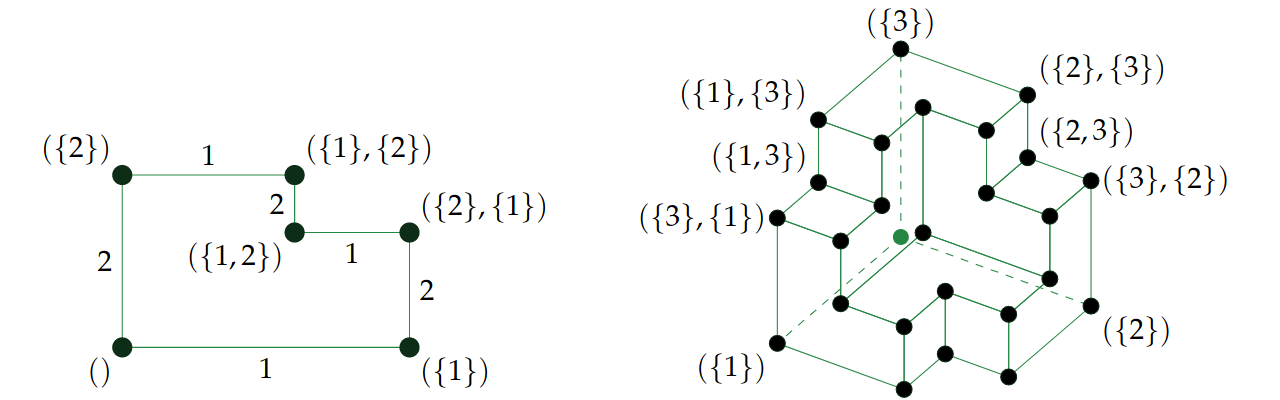Geometrically, the polytope has a simple description as being the set of all vectors$x \in \mathbb{R}^n$ such that, for all k, the kth smallest coordinate is in the interval [0, k]. It has volume$(n+1)^{n-1}$ and can tile the ambient space by translations.

But this doesn’t shed very much light on the combinatorial structure of the polytope, so it is useful to have a concrete description of the vertices, edges, and higher-dimensional faces. This was introduced in the paper, but the paper was chiefly concerned with the tensor rank of the determinant and therefore we did not delve too deeply into this.

As in the figure above, an ordered partial partition can be described by a list of disjoint subsets. For example, one of the OPPs of {1, …, 8} is:

[{3, 5}, {7}, {1, 4, 8}]

It is ordered because the order of the parts matters, and partial because some elements are allowed to be missing (in this case, 2 and 6). We can also draw this as a diagram where the parts are shown as layers, and all of the absent elements are present in a ‘ground level’ together with a sentinel value 0: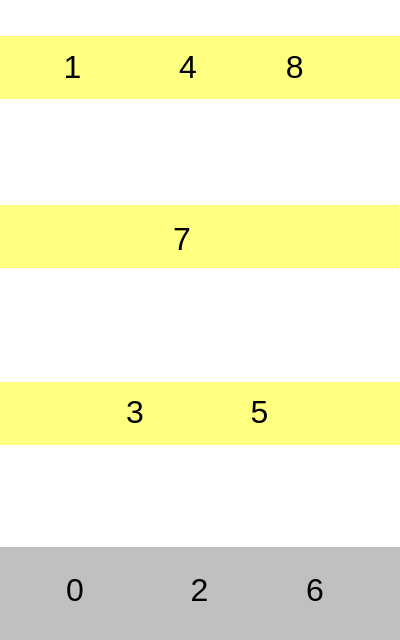Whilst such a diagram takes up a lot more space, it is more helpful for visualising how an OPP can be transformed into an adjacent OPP. The two allowed moves are:

• Taking a (nonzero) element that is the only occupant of its level (e.g. 7), and ‘merging it down’ into the next level (currently {3, 5}). The total number of levels decreases by 1.
• Taking a (nonzero) element that is not the only occupant of its level (e.g. 2), and ‘separating it up’ into its own new level. The total number of levels increases by 1.

(This description is different from, but equivalent to, the one in the paper: the paper omits the ‘ground level’ and therefore instead has elements disappear and reappear; here we include this ‘ground level’ explicitly to make some of the descriptions more concise.)

For a given element i ∈ {1, …, n}, exactly one of these two moves is possible (depending on whether or not it is the only occupant of its level), and the two moves are inverses of each other.

### The polytope and its face lattice

For a fixed n, we define a polytope whose vertices correspond to the OPPs of {1, …, n} and edges join pairs of OPPs if they can be interchanged by moving an element according to the aforementioned rules. Each vertex is incident with n edges, because from each OPP, we can move any of the n elements in exactly one way.

What about the higher-dimensional faces? A k-dimensional face is given by taking an OPP, allowing some size-k subset of the elements to be ‘movable’, and the remaining elements to be ‘immovable’. For example, we might make {2,3,7} movable elements, and the reachable configurations define a 3-dimensional face. Here we show the same OPP as before, but with the movable elements coloured in red: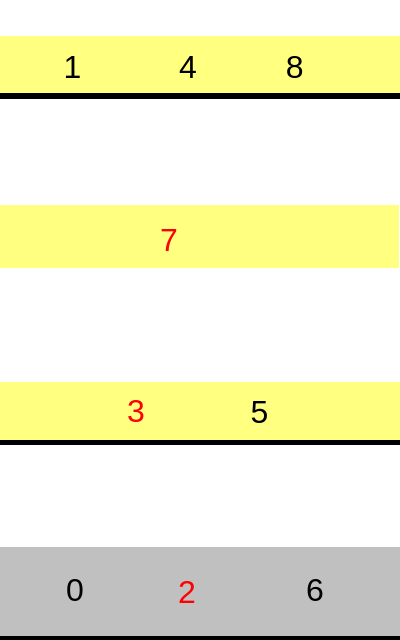We have also placed a solid black ‘floor’ on any level that contains an immovable element, because it is impossible for any elements to ever pass through those floors whilst subject to the rules we’ve described.

Which OPPs are reachable from this configuration? The element ‘2’ can stay on the ground level or move up into its own new level, so it has two possible locations. There are six possible configurations for the elements {3, 7}, depending on their relative orderings (3 possibilities) and whether or not at least one of them shares a level with ‘5’ (2 possibilities). Together, that means that this three-dimensional face has 12 vertices (where, by convention, we have omitted the contents of the ground level):

• [{3, 5, 7}, {1, 4, 8}]
• [{3, 5}, {7}, {1, 4, 8}]
• [{5}, {3}, {7}, {1, 4, 8}]
• [{5}, {3, 7}, {1, 4, 8}]
• [{5}, {7}, {3}, {1, 4, 8}]
• [{5, 7}, {3}, {1, 4, 8}]
• [{2}, {5, 7}, {3}, {1, 4, 8}]
• [{2}, {5}, {7}, {3}, {1, 4, 8}]
• [{2}, {5}, {3, 7}, {1, 4, 8}]
• [{2}, {5}, {3}, {7}, {1, 4, 8}]
• [{2}, {3, 5}, {7}, {1, 4, 8}]
• [{2}, {3, 5, 7}, {1, 4, 8}]

We have enumerated them in the order of a Hamiltonian cycle, making it apparent that these are indeed all reachable and therefore form a face of our polytope.

### Counting the faces

Note that any face has a unique ‘minimum-energy’ vertex: the ordered partial partition where we merge all movable elements down into the next level containing at least one immovable element. To count the faces in the whole polytope, it therefore suffices to count, for each ordered partial partition, the faces that have that particular OPP as their minimum-energy vertex.

A vertex is minimum-energy if and only if every non-ground level contains at least one immovable element. This means that for a particular ordered partial partition R, the set of movable elements must be chosen by taking some (possibly empty) proper subset of each non-ground level P ∈ R together with an arbitrary subset of the ground level. The generating function of faces with R as its minimum-energy vertex is therefore the polynomial:$(1 + x)^{n-m} \prod_{P \in R} \left( (1 + x)^{|P|} - x^{|P|} \right)$

where the coefficient of$x^k$ counts the number of k-dimensional faces with R as its minimum-energy vertex, and m is the number of non-ground elements in R.

To obtain the full face-generating function for the whole polytope, we just need to sum over all ordered partial partitions R. The number of such ordered partial partitions grows superexponentially, which is problematic. However, the polynomial doesn’t depend on the ordering of the parts or the specific choice of elements in each part, so we just need to sum over all partitions of each integer mn and multiply each polynomial that occurs by the number of ordered partial partitions corresponding to that particular integer partition.

Integer partitions grow subexponentially, so it is much more feasible to count them. Here is some Wolfram Mathematica code that uses this idea to compute the face-generating function for the whole polytope as a function of n: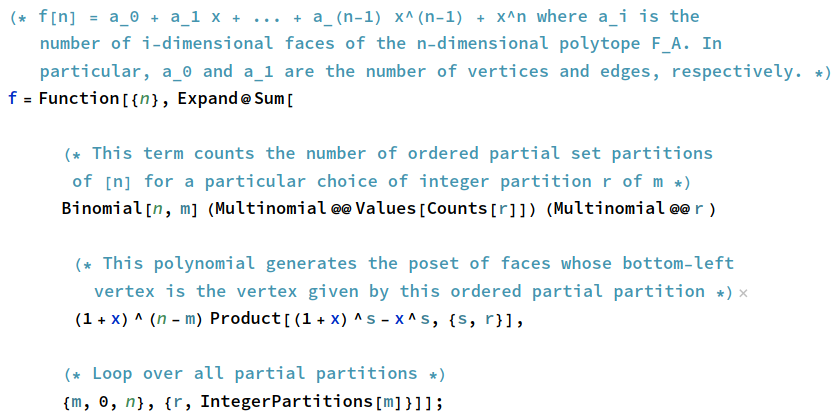It’s relatively quick to compute the face-generating function up to n = 30. Here are the values for n up to 8 dimensions: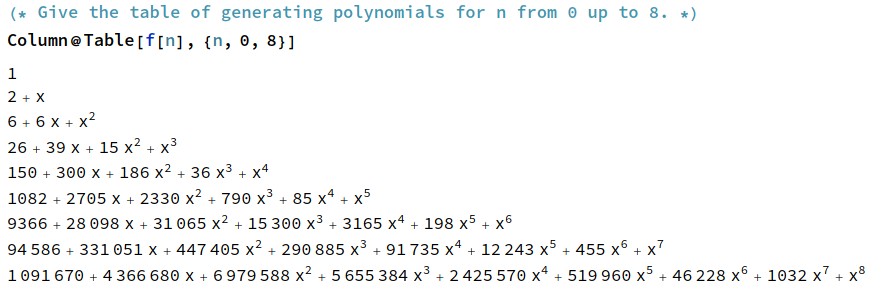The constant term counts the number of vertices, given by A000629 in the OEIS. The linear term counts the number of edges; it doesn’t appear to be in the OEIS, but by n-regularity, it’s just n/2 times the number of vertices.

The term in$x^{n-1}$ counts the codimension-1 faces, or facets, of the polytope. This sequence is A215149 in the OEIS, and has closed form$n(1 + 2^{n-1})$. We can interpret this geometrically: for each standard basis vector$e_i$, there are$2^{n-1}$ facets with outward normal$+e_i$ and one facet with outward normal$-e_i$; summing over all n of the standard basis vectors gives the result.

The polytopes start to get outrageously complicated for larger n. For example, when n = 30, there are more than 22 undecillion vertices, 342 undecillion edges, and 16 billion facets:Having a convenient way to compute the k-dimensional faces of the n-dimensional polytope in this family means that this could be submitted to the Encyclopedia of Combinatorial Polytope Sequences, which catalogues objects such as simplices, hypercubes, permutohedra, and associahedra.

### An infinite-dimensional polytope

As abstract polytopes, these are nested: every ordered partial partition of {1, …, n} is also an ordered partial partition of {1, …, n+1}. This means that we can take their union, and obtain an abstract infinite-dimensional polytope whose vertices are all finitely-supported ordered partial partitions of the positive integers.

This entry was posted in Uncategorized. Bookmark the permalink.

### 2 Responses to The ordered partial partition polytope

1.Андрей З. (@colt_browning) says:

Very interesting, thanks.

this could be submitted to the Encyclopedia of Combinatorial Polytope Sequences

Does this mean that you are going to do it, or this is a suggestion to readers to do it? 🙂

•apgoucher says:

Robin Houston has submitted it.